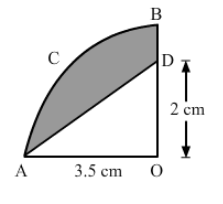# In the given figure, OABC is a quadrant of a circle of radius 3.5 cm with centre O.`
Question:

In the given figure, OABC is a quadrant of a circle of radius 3.5 cm with centre O. If OD = 2 cm, find the area of the shaded portion.Solution:

Area of the right-angled $\Delta \mathrm{COD}=\frac{1}{2} \times b \times h$

$=\frac{1}{2} \times 3.5 \times 2=3.5 \mathrm{~cm}^{2}$

Area of the sector $\mathrm{AOC}=\frac{\theta}{360} \times \pi \times r^{2}$

$=\frac{90}{360} \times \frac{22}{7} \times(3.5)^{2}$

$=9.625 \mathrm{~cm}^{2}$

Area of the shaded reaion = Area of the $\triangle \mathrm{COD}$ - Area of the sector $\mathrm{AOC}$

$=9.625-3.50$

$=6.125 \mathrm{~cm}^{2}$